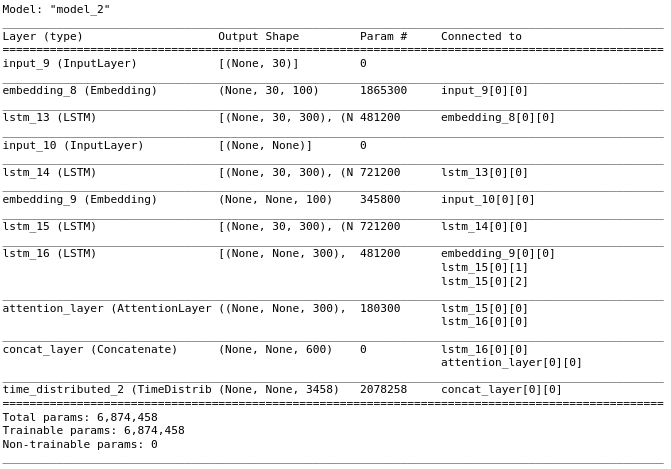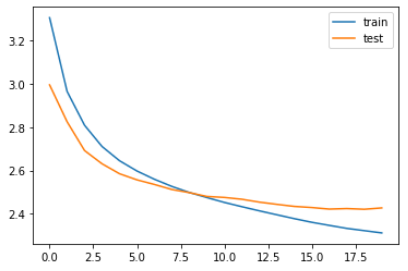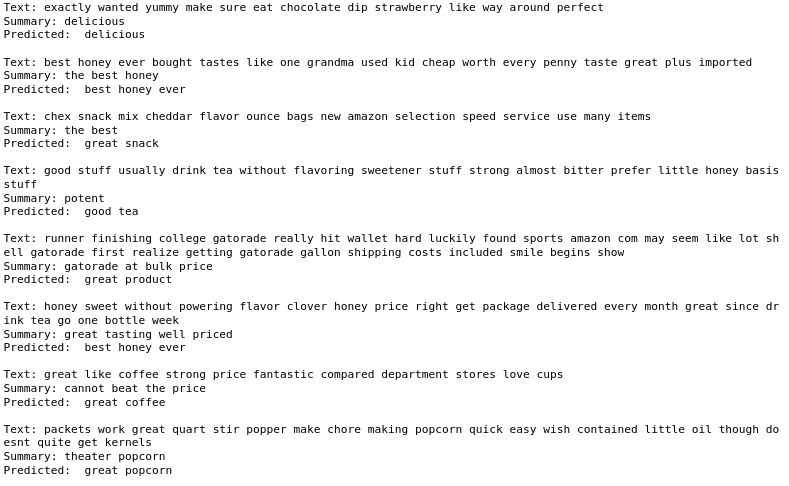There are many times when we are short of time and just want to get the job done as fast as possible. Sometimes when we are reading an article or looking at product reviews we don’t want to read the entire thing, but just the summary of it to understand what the large text wants to deliver.

It is really tedious to read an entire text and write a summary about it every time so why don’t we automate this task with the help of deep learning.

To summarize text using deep learning, there are two ways, one is Extractive Summarization where we rank the sentences based on their weight to the entire text and return the best ones, and the other is Abstractive Summarization where the model generates a completely new text that summarizes the given text.

In this article, we will be taking a look into Abstractive Summarization and discuss how it works.

Sequence-to-Sequence models

Abstractive Summarization uses sequence to sequence models which are also used in tasks like Machine translation, Name Entity Recognition, Image captioning, etc. Here we will be using the seq2seq model to generate a summary text from an original text. To put it simply what we are going to do is, use an encoder network to encode the original text and then use a decoder network to generate the summary by feeding the encoded data.

Import Packages

First, we will be importing all the packages required to build the model.

!Note below we imported AttentionLayer from a python file called ‘attention.py’ you can find that in the attachments to this article and we will discuss the attention layer later.

Python

Then we will load the dataset. Here we will be taking the first 100000 rows from the csv file. We have already preprocessed (converting to lowercase, removing special characters and numbers etc) the sentences in the dataset, you can find the code for it in the attachment section aswell.

Python

For our model we need to set the size of input and the size of output, to do so we can take a look into the distribution of lengths of the sentences or just calculate the average length of each sentence in both data[‘text’] and data[‘summary’].

Python

Which returns: 38.792962162284525 4.0105488336295005

Now that we get a rough idea of the lengths of sentences in data[‘text’] and data[‘summary’]. Let’s fix the max text length to 30 and max summary length to 8 (as there are some summaries longer than that so it’s better to have a max case for summary).

Python

We will add unique start and end tokens to each sentence in data[‘summary’], it will be useful for generating summaries as it will tell us when to start and when to stop the generation of summary.

Python

Which returns: ‘summstart good quality dog food summend’

Now we will split the data into training and validation, we will be using 10% of the data for validation and the rest for training.

Python

Tokenize Data

Now our dataset is ready, but the problem here is deep learning models do not take string inputs so we have to tokenize all of our sentences and convert them to numeric sequences. To do so we will be using the Tokenize method in the tensorflow.keras.preprocessing package. One more thing to care about is, when we are dealing with a large number of sentences there might be many words that are not used too often. So it’s unnecessary to increase the size of the tokenizer for those very rare words.

To deal with this we will set a minimum occurrence threshold and if any word has fewer occurrences than this, we will consider it as a rare word. Then we will take the total number of rare words and subtract it from the total number of unique words in our tokenizer.

Python

Using tokenizer to convert string sequences to integer sequences and adding padding to those sequences which do not match our fixed length. Also, we will calculate the size of the vocabulary of the tokenizer which will be used in the Embedding layer of our model.

Python

Delete all empty sequences (any sequences that has only start and end tokens are empty)

Python

Building and training the model

Now it’s time to build our model. Before that let’s understand the dataflow inside the model. From the following figure, we can see that our model has 2 networks one is an Encoder, and the other is a Decoder. The encoder takes our original long text sequence (tokenized) and returns its final hidden state and cell state which contains the information about our text sequence. The decoder then initializes its initial state with the output of the encoder network and performs a word-level sentence generation to generate the summary.

Encoder Model

The encoder model consists of LSTM layers, it takes the input sequence and computes the contextual information present in the input sequence. Its initial state can be taken as a zero vector or can be randomly initialized. It returns the hidden state and cell state which are used as the input to the decoder network.

Decoder Model

The decoder model also uses LSTM layers but its functionality is different, here the LSTM network predicts the next word by looking into the current word, a word-level sentence generator. The initial state of this network is the output of the encoder network.

Here the start and end are the unique tokens that we have added to each data[‘summary’] sentence earlier.

But this configuration is not enough to get a good performance. For long sequences the model will be unable to retain information. There are many ways to do so, but here we will use a technique called attention.

Usage of Attention

Simply putting the attention mechanism looks for a few parts of the sequence to predict a word rather than looking the whole sentence for it, which improves the information retention capacity of our model for long sequences.

Suppose we have a text: “I really like this product. It does what it says.”, which summarizes to “Good product”. The model will have to look for the entire sentence to generate the summary while with attention mechanism it maps specific parts, “like this product” in text with “good” in summary.

Let’s discuss about the two types of attention mechanisms, global attention, and local attention.

Global Attention

In a global attention model, the input from every encoder state and decoder state previous to the current state is taken into account to compute the output. From the following figure, the ‘Global align weights’ (a_t) are calculated using each encoder (blue blocks) states, and the previous decoder (red blocks) state (h_t). The context vector is calculated by taking the product of global align weights and each encoder steps. The result is then used to return the decoder output.

Local Attention

Here the model considers a few positions from the encoder and used to calculate the align weights (a_t). From the figure below, it can be referred that the single-aligned position (p_t) is found and then a window of words from the encoder along with the decoders states (h_t) is used to calculate align weights and context vector, which is then used to find the output of the decoder.

Encoder-Decoder model with attention

Python
Python

Which returns:Python

After the training is complete, we will save the weights of the model to our system.

Python

To load the model we can use

Python

Lets take a look at the loss and validation loss graph of our model during its trainingWe can see the loss of the model is decreasing with time whichc really great. Now its time to use our model to generate summary of texts. Before that let’s create a dictionary to convert integer tokens back to words and words to integers

Python

To generate summaries with the model that we just trained let’s build the inference model that will use the layers from our model and use the trained weights to generate a summary.

Python

Now we will define a function to generate encoded text using the encoder and then using that encoded text to generate the summary with the decoder. Here we will take advantage of the start and end tokens that we haved added to the data[‘summary’] earlier. We will stop generating once we hit the end token or we reach the max length for summary.

Python

To check how our model performs let’s display the original text, original summary, and predicted summary. For that, we need to define another two functions to convert the x_train and y_train (which we tokenized) to string sentences.

Python
Python

Which returns:From the output, you can clearly see that our model performs really well and summarizes the text that matches the context of given summaries. With that our Abstractive Text summarization model is complete. There are still a lot of ways it can be improved, by taking a large training dataset, trying different models like BERT, Bi-Directional LSTM etc.

Hope you enjoyed this blog and got to learn something new! Feel free to share your thoughts on this.

You can find the files used here from this